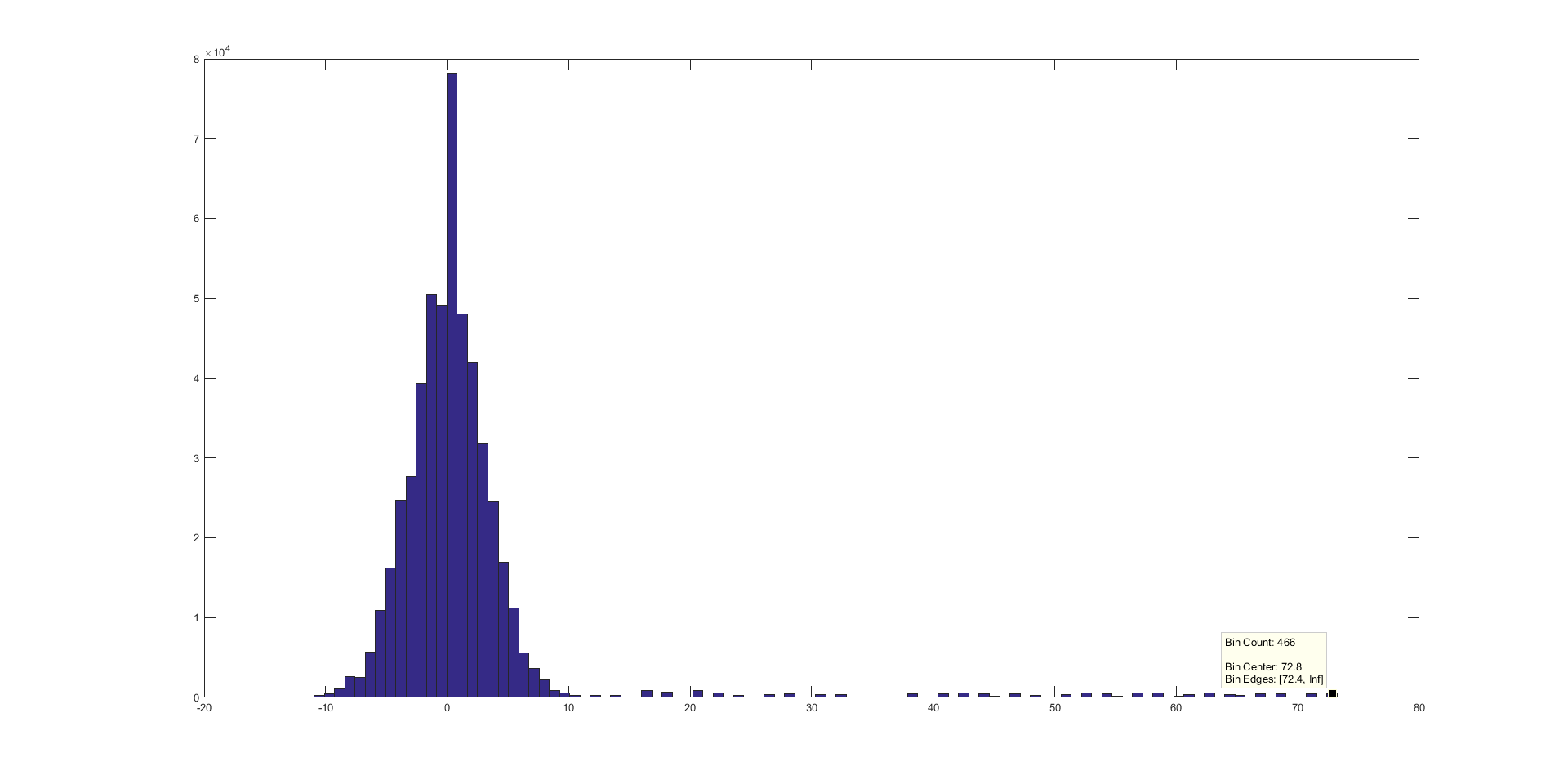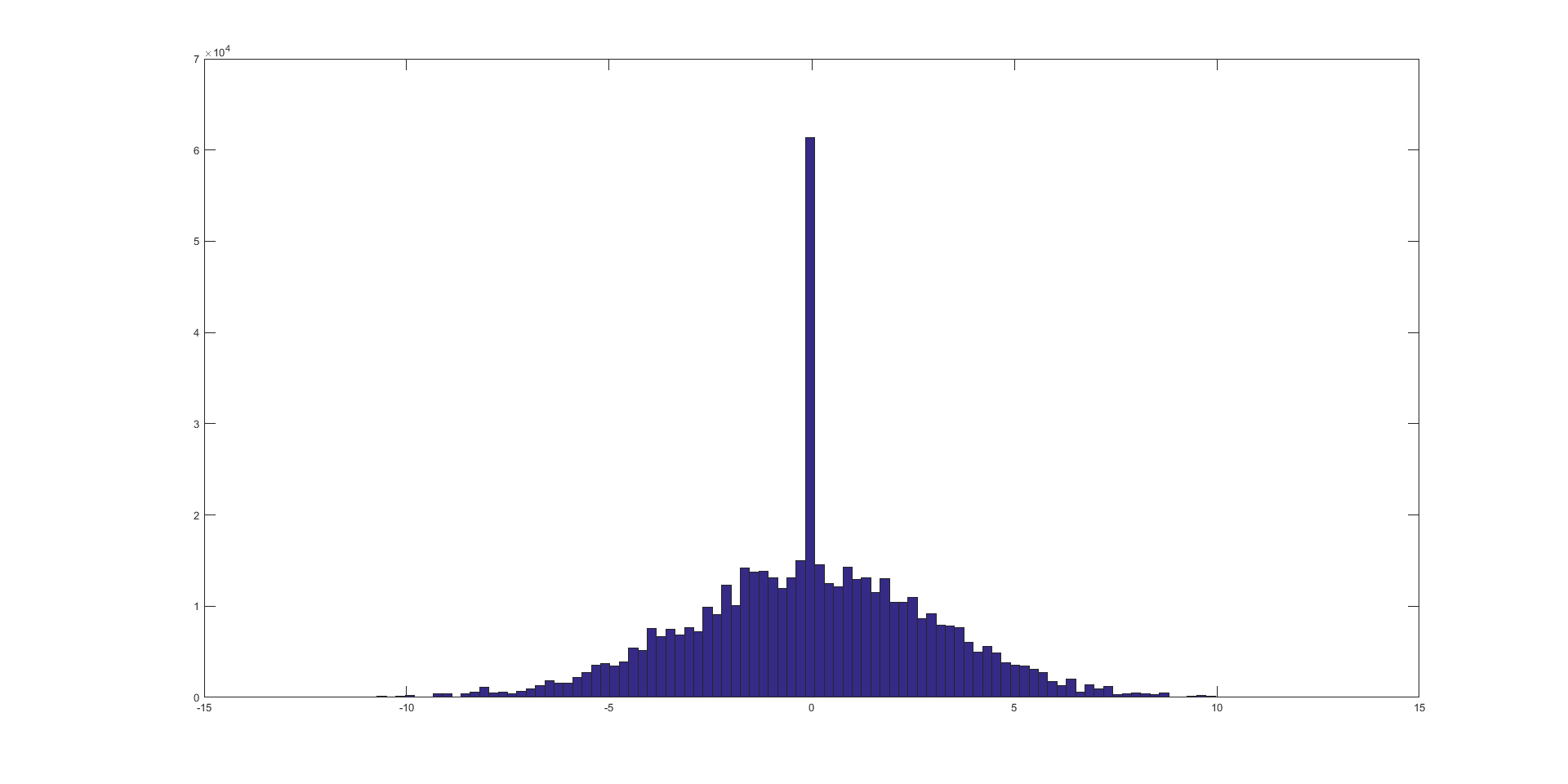# custom fix: bin & average "local" velocity, strange behavior

I’m working on a custom fix where my sample is binned (1D) into thin slices and some quantity is computed.
This quantitity also includes the neighbors of every atom.

For simplicity, let’s say its just the sum of all the atoms within each of these slices.

so at the end of every step the following is done.

void Myfix::dosome()
{
double **x = atom->x;
double **v = atom->v;

int *ilist,*jlist,*numneigh,**firstneigh;
int i,j,inum,jnum;

inum = list->inum;
ilist = list->ilist;
numneigh = list->numneigh;
firstneigh = list->firstneigh;

//These are my test-variables

double vcomx,vcomy,vcomz;
int count=0;
vcomx=vcomy=vcomz=0.0;

for(int ii = 0; ii < inum; ii++)
{
i=ilist[ii];
jlist = firstneigh[i];
jnum = numneigh[i];
if (mask[i] & groupbit)
{

//bin sample into thin slices

double xscale = (x[i] - domain->boxlo)/domain->xprd;
int ixnode = static_cast(xscale*nxnodes);

while (ixnode > nxnodes-1) ixnode -= 1;
while (ixnode < 0) ixnode += 1;

//subloop over all neighs
for(int jj=0;jj<jnum;jj++)
{
j=jlist[jj];

//just a testcase. Pick some slice. Happens to be on proc 0

if(ixnode==138)
{
vcomx+=v[j];
vcomy+=v[j];
vcomz+=v[j];
count+=1;
}
}

}

}

//Now output on proc 0
if(me==0)
fprintf(screen,“count:d,v:.4e\n”,
count,

sqrt( pow(vcomx,2.0)
+pow(vcomy,2.0)
+pow(vcomz,2.0)) );

}

Of course I’ve included all the necessary headers. And it runs. But the following is kind of weird:

If I run this with 3 procs I get a very different result for the magnitude of the summed velocity compared to a run on, let’s say 6 procs.
In both cases proc 0 happens to contain the same “slice” of the sample (nxnodes is the same for both scenarios)

For 3 procs, v turns out to be somewehere around 1e4, for 6 procs it’s around 1e7 (metal units)

But “count” is the same for both cases. It’s around 5e5. Should be good enough for some statistics (even though atoms sampled and counted multiple times)

The sample is equilibrated so the atomic velocities are the same on average. However, the more procs I use the larger the magnitude of the summed velocity.
I’m not saying that this quantitiy should turn out the same, but “v/count” should be very similar, shouldn’t it?
It isn’t. Since count is the same for both cases.

I’ve tried to find the bug on my own for hours now, but I guess it’s just too obvious for me to notice and I’m too tired anyway …
By the way, I’m using a cutoff of around 8 Angstrom and an EAM-potential for my metal-sample.

Can you tell me what I’m doing wrong here?

Best regards,
frank.

Are you requesting a full or a half neighbor list?

Axel

a full

int irequest = neighbor->request(this,instance_me);
neighbor->requests[irequest]->pair = 0;
neighbor->requests[irequest]->fix = 1;
neighbor->requests[irequest]->half = 0;
neighbor->requests[irequest]->full = 1;

A full neighbor list is good.

Next question: do you communicate ghost atom velocities?

Axel

I just plotted the histograms for the x-component of atomic velocity for all the neighbor-atoms at the first step in my chosen slice. As you can see, for the 6-proc case there are some ridiculously high velocities present.

Further I calculated the local temperatures from all the neighbor velocities and it seems that for 3 procs the result is incorrect as well. The temperature should be around 300 K while this procedure gives me something like 270-280 K on average (for 6 procs its around 1e6 K )That doesn’t answer my question. Which means that you are not likely to do it. That is consistent with the behavior you describe. You are probably reading random garbage data from uninitialized memory. You can confirm that by checking with valgrind’s memcheck tool.

Axel.

Greetings,

Try your code with the addition of turning on ghost velocity through comm_modify in your input script if you haven’t already.